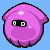# Science Quiz / Fun With 1234

Random Science or Math Quiz

## Can you name the order of the numbers 1-4 that complete the following equations?

#### bytobley  Plays Quiz Updated Mar 6, 2020

How to Play
Score
0/20
Timer
06:00
EquationOrder
_ ⁄ _ – _ + _ = 0
_ * _ – _ ⁄ _ = 1
_ – _ + _ * _ = 1
_ + _ ⁄ _ – _ = 1
_ + _ – _ ⁄ _ = 2
_ * _ ⁄ _ – _ = 2
_ * _ + _ – _ = 3
_ – _ ⁄ _ + _ = 3
_ – _ + _ ⁄ _ = 4
_ ⁄ _ + _ * _ = 5
EquationOrder
_ – _ * _ + _ = 5
_ + _ * _ – _ = 6
_ ⁄ _ * _ + _ = 7
_ * _ – _ + _ = 9
_ ⁄ _ * _ – _ = 10
_ + _ ⁄ _ * _ = 10
_ + _ * _ ⁄ _ = 11
_ * _ ⁄ _ + _ = 11
_ – _ + _ * _ = 13
_ ⁄ _ * _ + _ = 14

## From the Vault See Another

Four-Legged Critters
Science 90s
These animals seem pretty happy with the quadruped lifestyle.